Share

# Chemistry Science Paper 2 2015-2016 ICSE Class 10 Question Paper Solution

SubjectChemistry
Year2015 - 2016 (March)
Chemistry [Science Paper 2]
Marks: 80Date: 2016-03-18
Duration: 2h

Question 1 is Compulsory.

Attempt any four from Question 2 to Question 7

1
1.1

Metals are good _____________ (oxidizing agents / reducing agents) because they are electron __________ (acceptors / donors).

Concept: Metals and Nonmetals - Physical Properties - Metal
Chapter:

Electrovalent compounds have ________________ (high / low) melting points.

Concept: Concept of Electrovalent
Chapter: [0.02] Chemical Bonding

Higher the pH value of a solution, the more ____________ (acidic / alkaline) it is.

Concept: Position of the Metals (Alkali Metals and Alkaline Earth Metals) in the Periodic Table
Chapter:

_____________ (AgCl / PbCl2), a white precipitate is soluble in excess NH4OH

Concept: Formation and Colour of Hydroxide Precipitated for Solutions of Salts of Ca, Fe, Cu, Zn and Pb
Chapter: [0.04] Analytical Chemistry:- Use of Ammonium Hydroxide and Sodium Hydroxide

Conversion of ethane to ethane is an example of ____________ (hydration / hydrogenation).

Concept: Alcohols - Concept of Ethanol
Chapter: [0.09] Organic Chemistry
1.2

Choose the correct answer from the options given below

An element with the atomic number 19 will most likely combine chemically with the element whose atomic number is:

(A) 17

(B) 11

(C) 18

(D) 20

Concept: Periodicity on the Basis of Atomic Number for Elements
Chapter: [0.01] Periodic Properties and Variations of Properties:- Physical and Chemical

Choose the correct answer from the options given below :

The ratio between the number of molecules in 2g of hydrogen and 32g of oxygen is :

(A) 1 : 2

(B) 1:0.01

(C) 1:1

(D) 0.01:1 [Given that H = 1, O = 16]

Concept: Molecule - Concept of Molecule
Chapter: [0.03] Study of Acids, Bases and Salts

Choose the correct answer from the options given below :

The two main metals in Bronze are:

(A) Copper and zinc

(C) Copper and nickel

(D) Copper and tin

Concept: Metals and Nonmetals - Concept of Metals and Non-metals
Chapter:

Choose the correct answer from the options given below :

The particles present in strong electrolytes are :

(A) Only molecules

(B) Mainly ions

(C) Ions and molecules

(D) Only atoms

Concept: Electrolysis - Substances Containing Ions Only
Chapter:

Choose the correct answer from the options given below :

The aim of the Fountain experiment is to prove that :

(A) HCl turns blue litmus red

(B) HCl is denser than air

(C) HCl is highly soluble in water

(D) HCl fumes in moist air

Concept: Hydrogen Chloride - Density and Solubility of Hydrogen Chloride (Fountain Experiment)
Chapter: [0.083] Hydrogen Chloride
1.3

Write balanced chemical equations for Action of warm water on AIN.

Concept: Aqueous Solution of Ammonia
Chapter: [0.081] Ammonia

Write balanced chemical equations for Action of hot and concentrated Nitric acid on copper

Concept: Nitric Acid - Laboratory Method of Preparation of Nitric Acid from Potassium Nitrate Or Sodium Nitrate
Chapter: [0.08199999999999999] Nitric Acid

Write balanced chemical equations for Action of Hydrochloric acid on sodium bicarbonate.

Concept: Hydrogen Chloride - Preparation of Hydrogen Chloride from Sodium Chloride
Chapter: [0.083] Hydrogen Chloride

Write balanced chemical equations for Action of dilute Sulphuric acid on Sodium Sulphite

Concept: Sulphuric Acid - Behaviour as an Acid When Dilute
Chapter: [0.084] Sulphuric Acid

Write balanced chemical equations for Preparation of ethanol from Ethyl Chloride

Concept: Alcohols - Concept of Ethanol
Chapter: [0.09] Organic Chemistry
1.4

State your observations when Dilute Hydrochloric acid is added to Lead nitrate solution and the mixture is heated.

Concept: Hydrogen Chloride - Acidic Properties of Its Solution
Chapter: [0.083] Hydrogen Chloride

State your observations when Barium chloride solution is mixed with Sodium Sulphate Solution.

Concept: Formation and Colour of Hydroxide Precipitated for Solutions of Salts of Ca, Fe, Cu, Zn and Pb
Chapter: [0.04] Analytical Chemistry:- Use of Ammonium Hydroxide and Sodium Hydroxide

State your observations when Concentrated Sulphuric acid is added to Sugar Crystals

Concept: Sulphuric Acid - Oxidizing Agent When Concentrated
Chapter: [0.084] Sulphuric Acid

State your observations when Dilute Hydrochloric acid is added to Copper carbonate

Concept: Hydrogen Chloride - Acidic Properties of Its Solution
Chapter: [0.083] Hydrogen Chloride

State your observations when Dilute Hydrochloric acid is added to Sodium thiosulphate.

Concept: Hydrogen Chloride - Acidic Properties of Its Solution
Chapter: [0.083] Hydrogen Chloride
1.5

Identify the term/substance in the following:

The tendency of an atom to attract electrons to itself when combined in a compound.

Concept: Periodic Properties - Electronegativity
Chapter: [0.01] Periodic Properties and Variations of Properties:- Physical and Chemical

Identify the term/substance in the following:

The method used to separate ore from gangue by preferential wetting

Concept: Metals and Their Alloys - Common Ores of Iron, Aluminium and Zinc
Chapter: [0.07] Metallurgy

Identify the term/substance in the following:

The catalyst used in the conversion of ethyne to ethane.

Concept: Alcohols - Concept of Ethanol
Chapter: [0.09] Organic Chemistry

Identify the term/substance in the following:

The type of reactions alkenes undergo.

Concept: Organic Chemistry - Hydrocarbons - Alkenes,
Chapter: [0.09] Organic Chemistry

Identify the term/substance in the following:

The electrons present in the outermost shell of an atom.

Concept: Concept of Coordinate Bond
Chapter: [0.02] Chemical Bonding
1.6

A gas of mass 32 gms has a volume of 20 liters at S.T.P. Calculate the gram molecular weight of the gas.

Concept: Molar Volume of a Gas at S.T.P
Chapter: [0.05] Mole Concept and Stoichiometry

How much Is Calcium oxide formed when 82g of calcium nitrate is heated? Also, find the volume of nitrogen dioxide evolved :

2Ca(NO3)2 → 2CaO+4NO2+O2

(Ca = 40 , N = 14,O = 16)

Concept: Molar Volume of a Gas at S.T.P
Chapter: [0.05] Mole Concept and Stoichiometry
1.7

Match the salts given in Column I with their method of preparation given in Column II

 Column I Column II 1 Pb(NO3)2 from PbO A Simple displacement 2 MgCl2 from Mg B Titration 3 FeCl3 from Fe C Neutralization 4 NaNO3 from NaOH D Precipitation 5 ZnCO3 from ZnSO4 E Combination
Concept: Formation and Colour of Hydroxide Precipitated for Solutions of Salts of Ca, Fe, Cu, Zn and Pb
Chapter: [0.04] Analytical Chemistry:- Use of Ammonium Hydroxide and Sodium Hydroxide
1.8

Write the IUPAC names of the following: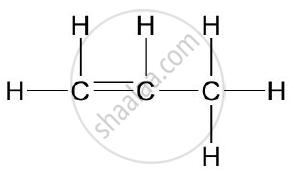Concept: Organic Chemistry - Simple Nomenclature
Chapter: [0.09] Organic Chemistry

Write the IUPAC names of the following: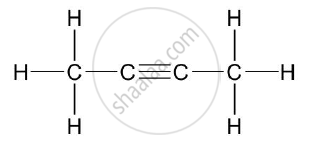Concept: Organic Chemistry - Simple Nomenclature
Chapter: [0.09] Organic Chemistry

Write the IUPAC names of the following: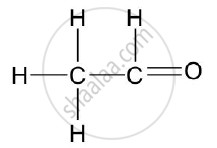Concept: Organic Chemistry - Simple Nomenclature
Chapter: [0.09] Organic Chemistry

Rewrite the following sentences by using the correct symbol > (greater than) or < (less than) in the blanks given

The ionization potential of potassium is _________________ that of sodium.

Concept: Periodic Properties - Ionisation Potential
Chapter: [0.01] Periodic Properties and Variations of Properties:- Physical and Chemical

Rewrite the following sentences by using the correct symbol > (greater than) or < (less than) in the blanks given

The electronegativity of iodine is ___________ that of Chlorine

Concept: Periodic Properties - Electronegativity
Chapter: [0.01] Periodic Properties and Variations of Properties:- Physical and Chemical
2
2.1

Use the letters only written in the Periodic Table given below to answer the questions that Follow :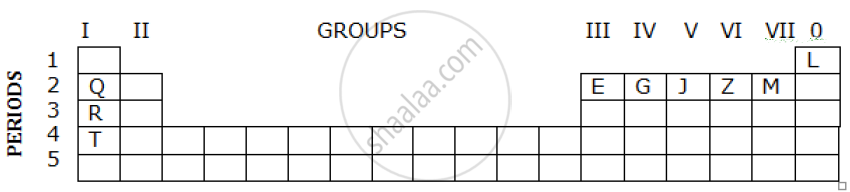1) State the number of valence electrons in atom J.

2) Which element shown forms ions with a single negative charge?

3)Which metallic element is more reactive than R?

4) Which element has its electrons arranged in four shells?

Concept: Periodicity on the Basis of Atomic Number for Elements
Chapter: [0.01] Periodic Properties and Variations of Properties:- Physical and Chemical
2.2 | Fill in the blanks by selecting the correct word from the brackets

If an element has a low ionization energy then it is likely to be ______________ (metallic / non metallic).

Concept: Metals and Nonmetals - Concept of Metals and Non-metals
Chapter:

If an element has seven electrons in its outermost shell then it is likely to have the ______________ (largest / smallest) atomic size among all the elements in the same period.

Concept: Periodic Properties - Atomic Size
Chapter: [0.01] Periodic Properties and Variations of Properties:- Physical and Chemical
2.3

The following table shows the electronic configuration of the elements W, X, Y, Z:

 Element w x y z Electronicconfigurations 2, 8, 1 2, 8, 7 2, 5 1

Answer the following questions based on the table above:

1) What type of Bond is formed between :

a) W and X

b) Y and Z

2) What is the formula of the compound formed between :

a) X and Z

b) W and X

Concept: Periodicity on the Basis of Atomic Number for Elements
Chapter: [0.01] Periodic Properties and Variations of Properties:- Physical and Chemical
3
3.1

Write a balanced chemical equation for Burning of ethane in the plentiful supply of air.

Concept: Alcohols - Concept of Ethanol
Chapter: [0.09] Organic Chemistry

Write a balanced chemical equations for Action of water on Calcium carbide

Concept: Organic Chemistry - Simple Nomenclature
Chapter: [0.09] Organic Chemistry

Write a balanced chemical equations for Heating of Ethanol at 170°C in the presence of conc. Sulphuric acid

Concept: Alcohols - Concept of Ethanol
Chapter: [0.09] Organic Chemistry
3.2

Give the structural formulae of 2-methylpropane.

Concept: Structure of Compounds with Single, Double and Triple Bonds
Chapter: [0.09] Organic Chemistry

Give the structural formulae of Ethanoic acid

Concept: Structure of Compounds with Single, Double and Triple Bonds
Chapter: [0.09] Organic Chemistry

Give the structural formulae of Butan – 2 -ol

Concept: Structure of Compounds with Single, Double and Triple Bonds
Chapter: [0.09] Organic Chemistry
3.3

The equation for the reaction when compound A is bubbled through bromine dissolved in carbon tetrachloride is as follows: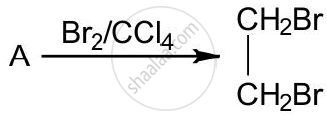1) Draw the structure of A.

2) State your observation during this reaction.

Concept: Concept of Organic Compounds
Chapter: [0.09] Organic Chemistry
3.4

Fill in the blanks using the appropriate words given below :

(Sulphur dioxide, nitrogen dioxide, Nitric oxide, Sulphuric acid)

Cold, dilute nitric acid reacts with copper to give ___________.

Concept: Nitric Acid as an Oxidizing Agent.
Chapter: [0.08199999999999999] Nitric Acid

Fill in the blanks using the appropriate words given below :

(Sulphur dioxide, nitrogen dioxide, Nitric oxide, Sulphuric acid)

Hot, concentrated nitric acid reacts with sulphur to form ______________.

Concept: Nitric Acid as an Oxidizing Agent.
Chapter: [0.08199999999999999] Nitric Acid
4
4.1

Identify the gas evolved and give the chemical test in the following cases

Dilute hydrochloric acid reacts with sodium sulphite.

Concept: Hydrogen Chloride - Preparation of Hydrogen Chloride from Sodium Chloride
Chapter: [0.083] Hydrogen Chloride

Identify the gas evolved and give the chemical test in the following cases

Dilute hydrochloric acid reacts with iron (II) sulphide.

Concept: Hydrogen Chloride - Preparation of Hydrogen Chloride from Sodium Chloride
Chapter: [0.083] Hydrogen Chloride
4.2

State your observations when ammonium hydroxide solution is added drop by drop and then in excess to each of the following solutions :
1) Copper sulphate solution

2) Zinc sulfate solution.

Concept: On Solution of Salts - Special Action of Ammonium Hydroxide on Solutions of Copper Salt
Chapter: [0.04] Analytical Chemistry:- Use of Ammonium Hydroxide and Sodium Hydroxide
4.3

Write equations for the reactions taking place at the two electrodes (mentioning clearly the name of the electrode) during the electrolysis of Acidified copper sulphate solution with copper electrodes

Concept: Electrolysis - Substances Containing Both Molecules and Ions
Chapter:

Write equations for the reactions taking place at the two electrodes (mentioning clearly the name of the electrode) during the electrolysis of Molten lead bromide with inert electrodes

Concept: Electrolysis - Substances Containing Both Molecules and Ions
Chapter:
4.4

Name the product formed at the anode during the electrolysis of acidified water using platinum electrodes.

Concept: Electrolysis - Elementary Study of the Migration of Ions
Chapter:

Name the metallic ions that should be present in the electrolyte when an article made of copper is to be electroplated with silver.

Concept: Applications of Electrolysis - Electroplating with Nickel and Silver
Chapter:
5
5.1

A gas cylinder contains 12 x 1024 molecules of oxygen gas.

If Avogadro’s number is 6 x 1023; Calculate:

1)The mass of oxygen present in the cylinder.

2) The volume of oxygen at S.T.P. present in the cylinder. [O = 16]

Chapter: [0.05] Mole Concept and Stoichiometry
5.2

A gaseous hydrocarbon contains 82.76% of carbon. Given that its vapor density is 29, find its molecular formula. [C = 12, H = 11]

Concept: Deduction of Simple (Empirical) and Molecular Formula from the Percentage Composition of a Compound
Chapter: [0.05] Mole Concept and Stoichiometry
5.3

The equation 4NH3 + 5O2 → 4NO + 6 H2O, represents the catalytic oxidation of ammonia. If 100 cm3 of ammonia is used calculate the volume of oxygen required to oxidize the ammonia completely

Concept: Catalytic Oxidation of Ammonia as the Source of Nitric Acid
Chapter: [0.081] Ammonia
5.4

By drawing an electron dot diagram show the formation of Ammonium ion [Atomic No.: N = 7 and H = 1]

Concept: Concept of Coordinate Bond
Chapter: [0.02] Chemical Bonding
6
6.1

Name the gas evolved when the following mixtures are heated:

Calcium hydroxide and Ammonium Chloride

Concept: Aqueous Solution of Ammonia
Chapter: [0.081] Ammonia

Name the gas evolved when the following mixtures are heated:

Sodium Nitrite and Ammonium Chloride

Concept: Nitric Acid - Laboratory Method of Preparation of Nitric Acid from Potassium Nitrate Or Sodium Nitrate
Chapter: [0.08199999999999999] Nitric Acid
6.2

Write balanced chemical equations for the following:

When the excess of ammonia is treated with chlorine.

Concept: Ammonia - Reactions with Hydrogen Chloride and with Hot Copper (Ii) Oxide and Chlorine
Chapter: [0.081] Ammonia

Write balanced chemical equations for the following:

An equation to illustrate the reducing nature of ammonia.

Concept: Ammonia - Reactions with Hydrogen Chloride and with Hot Copper (Ii) Oxide and Chlorine
Chapter: [0.081] Ammonia
6.3

A, B, C, and D summarize the properties of sulphuric acid depending on whether it is dilute or concentrated.

A = Typical acid property

B = Non volatile acid

C = Oxidizing agent

D = Dehydrating agent

Choose the property (A, B, C or D) depending on which is relevant to each of the following

1) Preparation of Hydrogen chloride gas

2) Preparation of Copper sulphate from copper oxide.

3) An action of conc. Sulphuric acid on Sulphur.

Concept: Sulphuric Acid - Behaviour as an Acid When Dilute
Chapter: [0.084] Sulphuric Acid
6.4

Give reasons why Sodium Chloride will conduct electricity only in the fused or aqueous solution state.

Concept: Electrolysis - Substances Containing Ions Only
Chapter:

Give reasons why In the electroplating of an article with silver, the electrolyte sodium argentocyanide solution is preferred over silver nitrate solution.

Concept: Applications of Electrolysis - Electroplating with Nickel and Silver
Chapter:

Give reasons why Although copper is a good conductor of electricity, it is a non-electrolyte.

Concept: Electrolysis - Electrolytes and Non-Electrolytes
Chapter: [0.06] Electrolysis
7
7.1

Name the solution used to react with Bauxite as a first step in obtaining pure aluminum oxide, in the Baeyer’s process

Concept: Metals and Their Alloys - Chemical Method - NaOH for Purifying Bauxite – Baeyer’s Process
Chapter: [0.07] Metallurgy

Write the equation for the reaction where the aluminum oxide for the electrolytic extraction of aluminum is obtained by heating aluminum hydroxide.

Concept: Metals and Their Alloys - Extraction of Aluminium
Chapter: [0.07] Metallurgy

Name the compound added to pure alumina to lower the fusion temperature during the electrolytic reduction of alumina.

Concept: Reduction of Metallic Oxides
Chapter: [0.07] Metallurgy

Write the equation for the reaction that occurs at the cathode during the extraction of aluminum by electrolysis.

Concept: Metals and Their Alloys - Extraction of Aluminium
Chapter: [0.07] Metallurgy

Explain why it is preferable to use a number of graphite electrodes as the anode instead of a single electrode, during the above electrolysis.

Concept: Electrolysis - Substances Containing Both Molecules and Ions
Chapter:
7.2

State what would you observe when Washing Soda Crystals are exposed to the atmosphere

Concept: General Properties of Salts:
Chapter: [0.03] Study of Acids, Bases and Salts

State what would you observe when The salt ferric chloride is exposed to the atmosphere

Concept: Salt - Types of Salts
Chapter: [0.03] Study of Acids, Bases and Salts
7.3

Identify the cations in the following case:

NaOH solution, when added to the Solution (A), gives a reddish brown precipitate

Concept: On Solution of Salts - Sodium Hydroxide on Ammonium Salts
Chapter: [0.04] Analytical Chemistry:- Use of Ammonium Hydroxide and Sodium Hydroxide

Identify the cations in the following case :

NH4OH Solution, when added to the Solution (B), gives white ppt which does not dissolve in excess.

Concept: Aqueous Solution of Ammonia
Chapter: [0.081] Ammonia

Identify the cations in the following case:

NaOH Solution, when added to Solution (C), gives white ppt which is insoluble in excess

Concept: Aqueous Solution of Ammonia
Chapter: [0.081] Ammonia

#### Request Question Paper

If you dont find a question paper, kindly write to us

View All Requests

#### Submit Question Paper

Help us maintain new question papers on Shaalaa.com, so we can continue to help students

only jpg, png and pdf files

## CISCE previous year question papers Class 10 Chemistry with solutions 2015 - 2016

CISCE Class 10 Chemistry question paper solution is key to score more marks in final exams. Students who have used our past year paper solution have significantly improved in speed and boosted their confidence to solve any question in the examination. Our CISCE Class 10 Chemistry question paper 2016 serve as a catalyst to prepare for your Chemistry board examination.
Previous year Question paper for CISCE Class 10 Chemistry-2016 is solved by experts. Solved question papers gives you the chance to check yourself after your mock test.
By referring the question paper Solutions for Chemistry, you can scale your preparation level and work on your weak areas. It will also help the candidates in developing the time-management skills. Practice makes perfect, and there is no better way to practice than to attempt previous year question paper solutions of CISCE Class 10.

How CISCE Class 10 Question Paper solutions Help Students ?
• Question paper solutions for Chemistry will helps students to prepare for exam.
• Question paper with answer will boost students confidence in exam time and also give you an idea About the important questions and topics to be prepared for the board exam.
• For finding solution of question papers no need to refer so multiple sources like textbook or guides.
S